# Welcome to the research group Differential Geometry and Geometric StructuresMembers & friends of the group in Jul 2021
photograph © by Narges Lali

Differential geometry has been a thriving area of research for more than 200 years, employing methods from analysis to investigate geometric problems. Typical questions involve the shape of smooth curves and surfaces and the geometry of manifolds and Lie groups. The field is at the core of theoretical physics and plays an important role in applications to engineering and design.

Finite and infinite geometric structures are ubiquitous in mathematics. Their investigation is often intimately related to other areas, such as algebra, combinatorics or computer science.

These two aspects of geometric research stimulate and inform each other, for example, in the area of "discrete differential geometry", which is particularly well suited for computer aided shape design.

# Gallery of some research interests and projects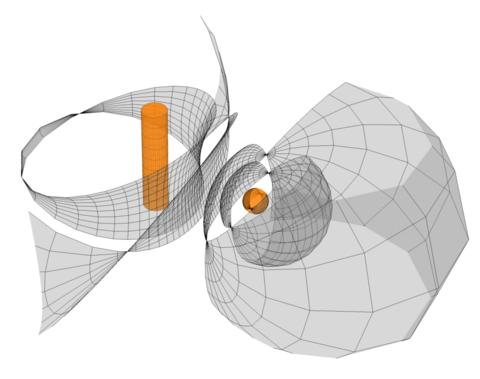Cyclic coordinate systems: an integrable discretization in terms of a discrete flat connection is discussed. Examples include systems with discrete flat fronts or with Dupin cyclides as coordinate surfaces (Hertrich-Jeromin, Szewieczek)We study surfaces with a family of spherical curvature lines by evolving an initial spherical curve through Lie sphere transformations, e.g., the Wente torus (Cho, Pember, Szewieczek)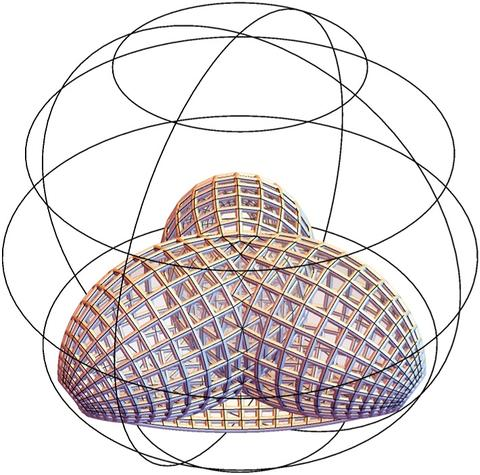Discrete Weierstrass-type representations are known for a wide variety of discrete surfaces classes. In this project, we describe them in a unified manner, in terms of the Omega-dual transformation applied to to a prescribed Gauss map. (Pember, Polly, Yasumoto)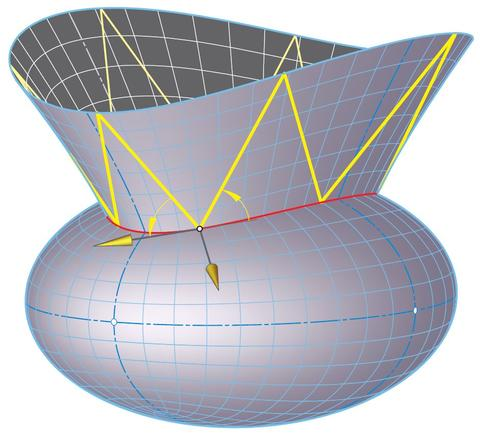Billiards: The research addresses invariants of trajectories of a mass point in an ellipse with ideal physical reflections in the boundary. Henrici's flexible hyperboloid paves the way to transitions between isometric billiards in ellipses and ellipsoids (Stachel).Spreads and Parallelisms: The topic of our research are connections among spreads and parallelisms of projective spaces with areas like the geometry of field extensions, topological geometry, kinematic spaces, translation planes or flocks of quadrics. (Havlicek)This is a surface of (hyperbolic) rotation in hyperbolic space that has constant Gauss curvature, a recent classification project. (Hertrich-Jeromin, Pember, Polly)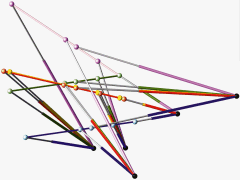Singularity Closeness of Stewart-Gough Platforms: This project is devoted to evaluating the closeness of Stewart-Gough platforms to singularities. (Nawratil)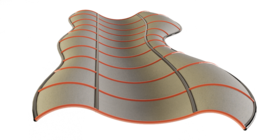Geometric shape generation: We aim to understand geometric methods to generate and design (geometric) shapes, e.g., shape generation by means of representation formulae, by transformations, kinematic generation methods, etc. (Hertrich-Jeromin, Fig Lara Miro)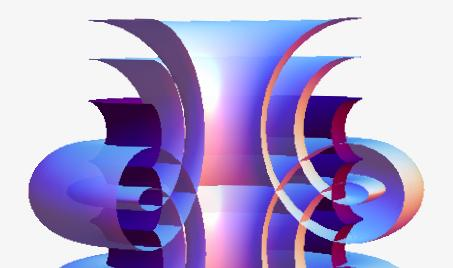Non-rigidity and Symmetry breaking: We investigate relations between non-rigidity and symmetry breaking, in particular, whether deformability of a surface or object in more than one way invariably leads to symmetry breaking. (Fuchs, Hertrich-Jeromin, Pember, Fig Mundilova)Affine Differential Geometry: In affine differential geometry a main point of research is the investigation of special surfaces in three dimensional affine space. (Manhart)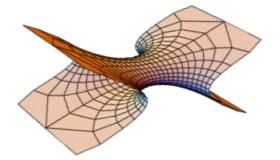Transformations & Singularities: We aim to understand how transformations of particular surfaces behave (or fail to behave) at singularities, and to study how those transformations create (or annihilate) singularities. The figure shows the isothermic dual of an ellipsoid, which is an affine image of a minimal Scherk tower. (Hertrich-Jeromin)Stewart Gough Platforms with Self-Motions: The main aim of the project is the systematic determination, investigation and classification of Steward-Gough platforms with self-motions. (Nawratil)

# News

27 Oct 2021: Geometry seminar 15:00, ZS1
Kostiantyn Drach (IST Austria): Reversing the classical inequalities under curvature constraints

## Abstract

A convex body $K$ is called uniformly convex if all the principal curvatures at every point along its boundary are bounded by a given constant lambda either above (lambda-concave bodies), or below (lambda-convex bodies). We allow the boundary of $K$ to be non-smooth, in which case the bounds on the principal curvatures are defined in the barrier sense, and thus the definition of lambda-convex/concave bodies makes sense in a variety of discrete settings. The intersection of finitely many balls of radius 1 is an example of a 1-convex body, while the convex hull of finitely many balls of radius 1 is an example of a 1-concave body.

Under uniform convexity assumption, for convex bodies of, say, given volume, there are non-trivial upper and lower bounds for various functionals, such as the surface area, in-, and outer-radius, diameter, width, meanwidth, etc. The bound in one direction usually constitutes the classical inequality: for example, the lower bound for the surface area is the isoperimetric inequality. The bound in another direction becomes a well-posed and in many cases highly non-trivial reverse optimization problem. In the talk, we will give an overview of the results and open questions on the reverse optimization problems under curvature constraints in various ambient spaces.

13 Oct 2021: Geometry seminar 15:00, ZS 1
Matty Van-Son (TU Wien): Geometry and Markov numbers

## Abstract

We discuss the history of Markov numbers, which are solutions to the equation $x^2+y^2+z^2=3xyz$. These solutions can be arranged to form a tree, and we show that similar trees of $SL(2,Z)$ matrices, quadratic forms, and sequences of positive integers relate very closely to Markov numbers. We use the tree structure of sequences, along with a geometric property of the minimal value of forms at integer points, to propose an extension to Markov numbers.

This is a joint work with Oleg Karpenkov (University of Liverpool).

18 Jun 2021 (Fri!): Geometry seminar
Graham Andrew Smith (Federal University of Rio de Janeiro): On the Weyl problem in Minkowski space

## Abstract

We show how the work of Trapani & Valli may be applied to solve the Weyl problem in Minkowski space.

This work is available on arXiv at https://arxiv.org/abs/2005.01137.

02 Jun 2021: Geometry seminar
Sergey Tabachnikov (Penn State University): Variations on the Tait-Kneser theorem

## Abstract

The Tait-Kneser theorem, first demonstrated by Peter G. Tait in 1896, states that the osculating circles along a plane curve with monotone non-vanishing curvature are pairwise disjoint and nested. I shall present Tait's proof and discuss variations on this result. For example, the osculating circles can be replaced by the osculating Hooke and Kepler conics along a plane curve; the proof uses the Lorentzian geometry of the space of these conics. I shall also present a version of this theorem for the graphs of Taylor polynomials of even degrees of a smooth function.
16-18 Sep 2019: Conference
ISHM2019: Integrable systems and harmonic maps, Vienna University of Technology
01 Feb 2019: Web site
The catalogues of our planar/spatial kinematic models are online